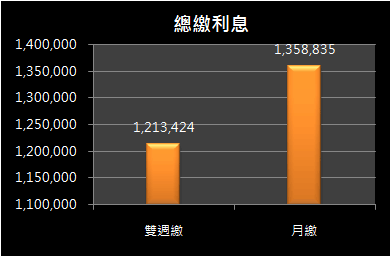# 基本概念# 並未佔到便宜

=NPER(2.5%/12,-28703,5000000)
=216.6期 (18年1個月)

=216.6*(28703)-5000000 = 1,217,070

# 雙週繳攤還表

=PMT(2.5%/12,240,-5000000)
=26,495

=NPER((2.5%/365)*14,-26495/2,5000000)
=468.77

=PMT((2.5%/365)*14, 470, -5000000)
=13,220

# 結論

閱讀文章‎ > ‎貸款規劃文章列表‎ > ‎貸款規劃‎ > ‎                                                                                                        怪老子理財首頁